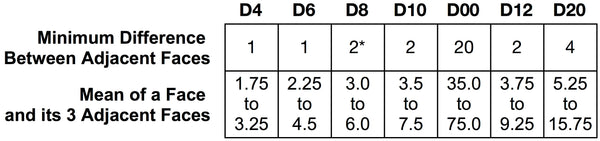# Mathematically Balanced

## Flying Horseduck Sixty Series

#### Rules for Numbering Layouts

Our numbering layouts are mathematically balanced to do justice to our precision milled dice. The painstaking process that required approximately 100 hours of work over dozens of late nights was worth the result of dice that are as fair and numerically elegant as they are pretty. The numbering layouts of our dice are bound by the following rules.

#### Rules for all dice:

1. Faces with the highest value on a die are each always exactly opposite a face with the lowest value
2. The sum of the five faces of any pentagon on a die is within 1 of the sum of any other pentagon (all equal is not possible)
3. Pentagons directly opposite each other on the die have equal sums
4. Highest and lowest values are spread across the die as evenly as practical

#### Rules Specific to Each Die:#### Notes and Definitions:

• The term "pentagon" is used to collectively refer to 5 faces that completely surround a vertex
• The term "opposite" means the other side of the die; if a die is resting on a face, the opposite face is up
• The term "adjacent" refers to two faces which share an edge
• On the D10 "0" is the value 10 and is considered the highest value on the die
• On the D00 "00" is the value 100 and is considered the highest value on the die
• On the D8 the re-roll faces are considered to have the value 4.5, which is the average roll of a D8
• * Re-roll faces are ignored in the "Difference Between Adjacent Faces" calculations